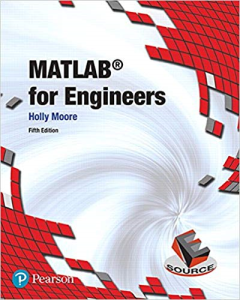# MATLAB for Engineers (5th Edition) 5th Edition

PLDZ-3 In Stock
\$ 5.00 USD
Description

Start at the beginning to introduce your students to MATLAB

MATLAB® For Engineers introduces  students the MATLAB coding language. Developed out of Moore’s experience teaching MATLAB and other languages, the text meets students at their level of mathematical and computer sophistication. Starting with basic algebra, the book shows how MATLAB can be used to solve a wide range of engineering problems. Examples drawn from concepts introduced in early chemistry and physics classes and freshman and sophomore engineering classes stick to a consistent problem-solving methodology.

Students reading this text should have an understanding of college-level algebra and basic trigonometry. The text includes brief backgrounds when introducing new subjects like statistics and matrix algebra. Sections on calculus and differential equations are introduced near the end and can be used for additional reading material for students with more advanced mathematical backgrounds.

Recent Reviews Write a Review
0 0 0 0 reviews